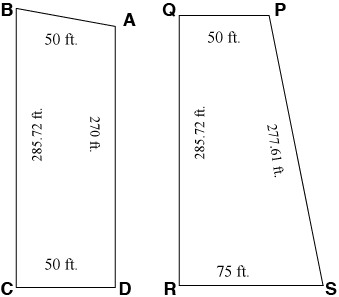SEARCH HOMEMath Central Quandaries & QueriesI have two lots which I am trying to calculate the square footage of one lot is 50 x 285.72 x 50 x 270 2nd lot is 50 x 285.72 x 50 x 277.61 Any help would be appreciated. The answers would be GREATLY appreciated. Thank you. LindaLinda,

I need more than the lengths of the sides to determine the area. I need to know the shape of the lot, either by telling me the measure of one of the angles or the length of a diagonal. Many different lots with different shapes and different areas could have these side lengths.

mathcentral.uregina.ca/QQ/database/QQ.09.03/dave1.html

Penny

Linda wrote back:

Hi
Thank you for your response and help with this. No one around here seems to be able to get me an answer. I have had all types.

The first lot is basically a rectangle with one side a little longer than the other.

The second lot is 50 on the top and 75 on the bottom with the left line running straight and the right line at a angle

Hope this helps
I am attaching a file with the drawings so you can see. Hope you can open it.

Linda,

The diagrams you sent do help but I am still not confident that I can find the area exactly. For example for the diagram on the left it looks like the angle at C and the angle at D are both right angles. This can't be true since if they were both right angles then the side from A to B would be longer than 50 ft. I will estimate the area based on the diagram but it really is an estimate.I am going to assume that the sides BC and DA are parallel. In that case ABCD is a trapezoid and the area of a trapezoid is given by

Area = the average of the lengths of the parallel sides, times the distance between the parallel sides.

In the case of ABCD this is

area = (270 + 285.72)/250 = 13893 square feet.

You can use the same technique for PQRS if you assume, as indicated by the diagram, that PQ and RS are parallel and the distance between then is 75 ft.

I hope this helps,
PennyMath Central is supported by the University of Regina and The Pacific Institute for the Mathematical Sciences.